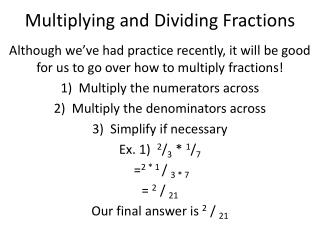DownloadDownload PresentationMultiplying and Dividing Fractions

# Multiplying and Dividing Fractions

Télécharger la présentation## Multiplying and Dividing Fractions

- - - - - - - - - - - - - - - - - - - - - - - - - - - E N D - - - - - - - - - - - - - - - - - - - - - - - - - - -
##### Presentation Transcript

1. Multiplying and Dividing Fractions Although we’ve had practice recently, it will be good for us to go over how to multiply fractions! Multiply the numerators across Multiply the denominators across Simplify if necessary Ex. 1) 2/3 * 1/7 =2 * 1 / 3 * 7 = 2 / 21 Our final answer is 2 / 21

2. We Should Try One Together! Ex. 2) 4/ 5 * 7 / 8 Multiply the numerators across Multiply the denominators across Simplify if necessary

3. Work with your Partners on a Few Ex. 3) 3/4 * 4/5 Ex. 4) 7/9 * 2/ 14 Ex. 5) 2/8 * 3/6 Ex. 6) 5/7 * 2/15

4. Dividing fractions Dividing fractions is a three- step process Find the reciprocal of the second fraction Multiply the numerators across, then multiply the denominators across Simplify if necessary

5. Dividing fractions Ex. 7) 1/3 ÷ 1/4 Find the reciprocal of the second fraction The reciprocal of 1/4 is 4/1 2) Multiply the numerators across, then multiply the denominators across 1/3* 4/1 = 4/3 Simplify if necessary Nothing to simplify, so the answer is 4/3

6. Dividing fractions (cont.) Ex. 8) 2/5÷ 4/15 Ex. 9) 7/9÷ 7/3